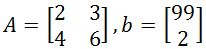# Solving linear systems using 2 x 2 inverse matrices

### Solving linear systems using 2 x 2 inverse matrices

Now that we learned how to solve linear systems with Gaussian Elimination and Cramer's Rule, we are going to use a different method. This method involves using 2 x 2 inverse matrices. To solve the linear system, we find the inverse of the 2 x 2 coefficient matrix (by using either row matrix operation or the formula) and multiply it with the answer column. Multiplying them would result in a column matrix, and the entries in the column matrix will give you a unique solution to the linear system.

#### Lessons

Back then we learned that the linear system
$1x+2y=3$
$4x+5y=6$

Can be represented as the matrix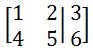Now we can actually represent this in another way without the variables disappearing, which is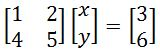Now let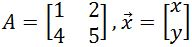, and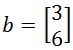. Then we can shorten the equation to be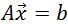.

Now multiplying both sides of the equation by $A^{-1}$ will give us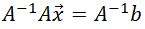We know that $A^{-1} A=I$, so then our equation becomes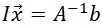.

We also know that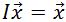, and so our final equation is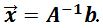With this equation, we can solve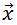(which has the variable $x$ and $y$) simply by finding the inverse of $A$, and multiplying it by $b$.
• Introduction
Solving linear systems using inverse matrices overview

• 1.
Solving the system of equations using inverse matrices
You are given $A$ and $b$. Knowing that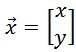, solve the following linear systems by finding the inverse matrices and using the equation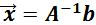.
a)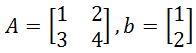b)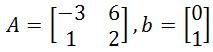c)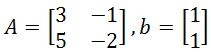d)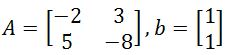e)